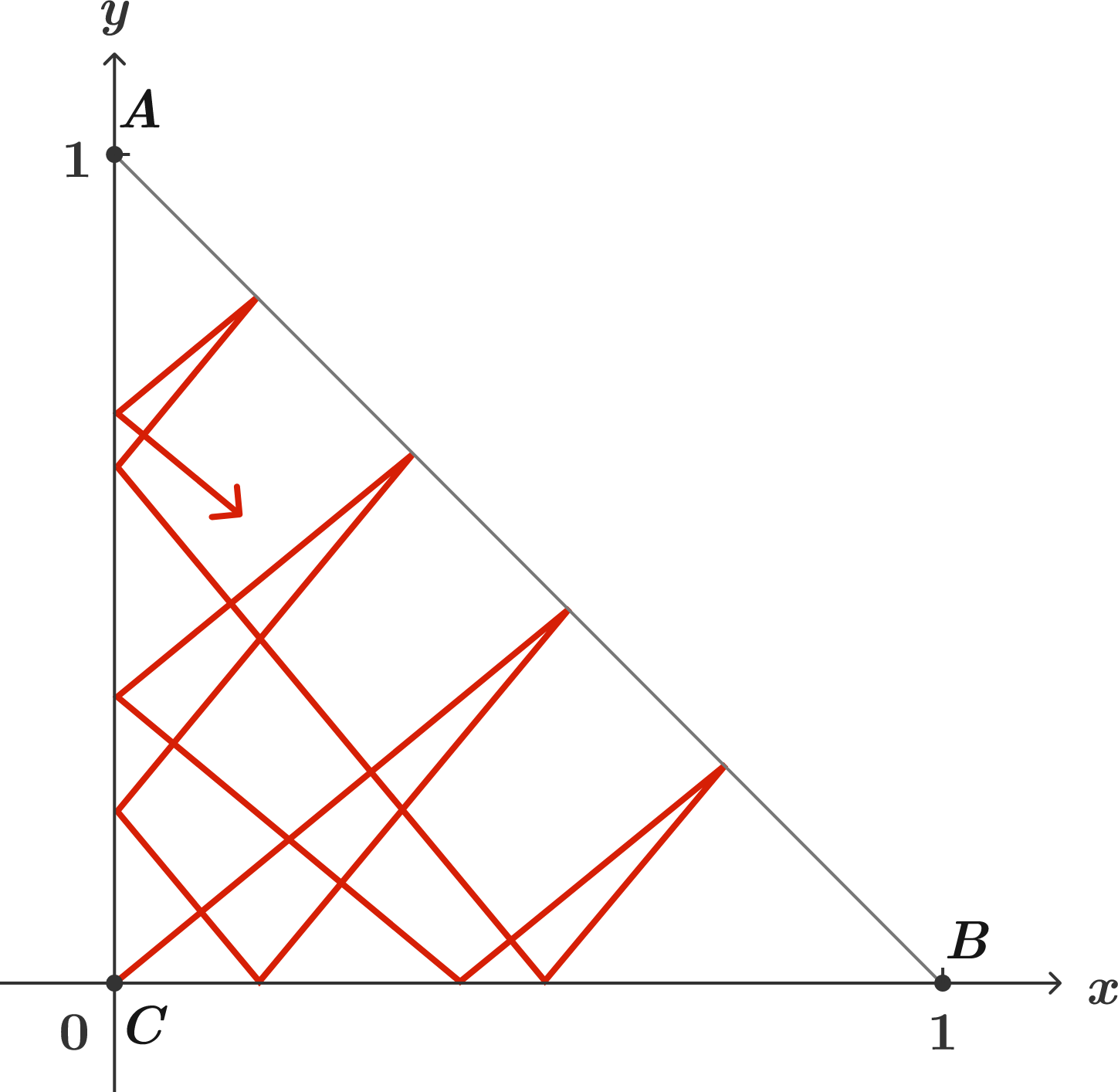# A lot of reflections

Geometry Level 2Triangle $ABC$ has vertices $A = (0,1),$ $B=(1,0),$ and $C=(0,0)$ in the coordinate plane.

The insides of the sides are lined with mirrors, and then a laser beam is fired from the origin with a slope of $\frac{314}{379}.$

Which corner of the triangle will the laser beam hit first?

×